# Time Averaging Techniques

There are a variety of techniques available for calculating a time average of a time series, like Pyth’s aggregate price. They each present tradeoffs in terms of complexity, sensitivity to outliers, and implementation cost.

When considering an approach for averaging Pyth data, we would like it to have the following properties:

1. Simplicity — generally speaking, the simpler something is, the more likely it is to behave as expected, and the more robust it will be to unpredictable conditions. The obvious tradeoff here is that something too simple may not have all of the other desired properties on our list. As Einstein famously said, “Everything should be made as simple as possible, but not simpler.
2. Robustness — we would like our averaging algorithm robust against messy data. One of Pyth’s innovations is that the aggregation process produces both an aggregate price and a confidence interval at each time slot. A robust averaging algorithm will make use of that confidence measure and not allow the average to be impacted by samples with outlier prices and wide confidence intervals. A robust averaging algorithm must also handle gaps and missing data in the time series.
3. Computational Efficiency — we would like our averaging algorithm to have small computational requirements on each price update and small storage requirements.

# Simple Moving Average (SMA)

Probably the simplest technique that most people will be familiar with is the Simple Moving Average or SMA. For example, to calculate a 1hr SMA, you keep track of all prices in the last 1hr window and average them. As time steps forward, old prices drop out of the back of the window, and new prices enter the front of the window.

While simple to understand, the SMA has a few drawbacks. The main one is that it requires you to store all of the prices within the averaging window, which in the case of a high-frequency data feed like Pyth, averaging windows of hours can turn into tens of thousands of numbers.

In its simplest form, it is also not robust to outliers with wide confidence or gaps of missing data. To add robustness through confidence weighting and handling of missing samples, confidence and timestamps for each sample have to be stored, tripling the already large storage requirements.

# Exponential Moving Average (EMA)

Another averaging method commonly used in finance and trading is the Exponential Moving Average or EMA. In an EMA, the most recent samples receive the most weight, and samples further back in time get exponentially less weight the farther in the past they are. For a 1hr EMA, the samples 1-hour in the past get 1/2 the weighting, samples 2-hours in the past get 1/4 of the weighting, 3 hours in the past get 1/8 of the weighting, etc.

Here is a plot showing the weighting of samples in an EMA compared to the weighting of samples in an SMA.

While conceptually not as simple as an SMA, the EMA has a particularly simple implementation for streaming applications such as Pyth. The exponential weighting method allows the entire history of prices and weights to be represented by a single number. To update the EMA when a new price comes in, the old average is decayed by an exponential weighting, and the new sample is added to it. With a storage footprint of a single number and a simple update rule, It is hard to beat an EMA for computational efficiency.

# Pyth’s Averaging Method

Pyth’s averaging method is a variation of an EMA that adds robustness. We preferred an EMA over an SMA because its computational efficiency is a good fit for on-chain applications. However, a basic EMA does not account for confidence intervals or missing data. Therefore, the current Pyth averaging method is a slot-weighted, inverse confidence-weighted exponential moving average of the aggregate price.

Inverse Confidence Weighted

Each aggregate price sample is also weighted by 1/Confidence in the EMA calculation. If there are samples that are outlier aggregate prices with very wide confidence intervals, we would like the average not to be impacted by those outlier aggregate prices. Weighting each sample by 1/Confidence lets the EMA give more weight to samples with tight confidence and ignore samples with very wide confidence. Below is an example of an outlier aggregate price with a wide confidence interval. Notice how the average using inverse confidence weighting does not get pulled up by the outlier sample while the uniform weighted average does.

Slot Weighted

The Pyth EMA uses the Solana slot number to measure the passage of time. The averaging period is 5921 slots, corresponding to approximately 1 hour on Solana mainnet.

What’s in a Name?

The average price has been called “TWAP” in the code, documentation, and the Pyth website. We plan on changing the name from TWAP to EMA Price (and TWAC to EMA Confidence) to avoid confusion in the wider Pyth community. Many of the folks involved in the original Pyth design and development come from the world of high-frequency trading, where moving averages are almost always implemented as EMAs since computational efficiency is so crucial (as it also is in scalable on-chain applications). We used the name TWAP to indicate that the average was time-weighted, as opposed to volume-weighted, volatility-weighted, or any number of other weighting methods often employed in trading systems. We plan to change the naming convention we use going forward to more accurately convey how the averaging is done.

# Confidence in the EMA

On each individual slot, the confidence represents the uncertainty in the aggregate price. When using the aggregate price, consumer applications can make use of the price and confidence in combination to take conservative actions that reflect the uncertainty in the price (for example: originate loans based on price — 3confidence but liquidate loans based on price + 3confidence).

Since the aggregate prices each have some uncertainty associated with them, the EMA of these prices also has some uncertainty associated with it. Pyth reports a confidence interval on the EMA that can be used by consumer applications in the same way they use the confidence of the aggregate price. This confidence used to be known as the TWAC, but will be renamed to EMA Confidence to reduce confusion.

Calculating the confidence in an EMA of prices from the confidence of the individual prices requires us to make some assumptions about the correlation of errors in the prices from slot to slot. If the errors were completely uncorrelated, then for each slot, we would take the square of the confidence as the variance in that slot and calculate the variance of the EMA to be the EMA of those variances, and then take the square root of the EMA variance to get confidence in the EMA.

However, the uncorrelated errors assumption is inaccurate in some cases. For example, consider a period of time where the price is constant, and the confidence represents the bid-ask spread of the market. In this case, the confidence in the EMA shouldn’t average down to a smaller value with more and more identical price samples. We have repeated measurements of the same market price and uncertainty here, not independent ones with uncorrelated uncertainties. Similarly, in cases where there are outlier samples with wide confidences, the underlying cause is likely to persist for some period of time and potentially cause many samples to have correlated prices and uncertainties. Treating them as uncorrelated could dramatically underestimate the width of the true confidence in the EMA.

The other extreme would be to assume that all the samples are perfectly correlated, and then the confidence in the EMA would be the EMA of the confidence of each slot. Now this is almost certainly an overestimate of the width of the true confidence in the EMA for long averaging periods, as the errors in prices are not likely to be correlated on the timescales of tens of minutes. The truth lies somewhere between these two extreme limits, but there is no clear way to calculate it simply.

Underestimating the confidence width in the EMA could be very bad, causing consumers to have more faith in the precision of the EMA than is warranted. Overestimating the confidence width is a “safer” choice and the one that Pyth follows. While an overestimate, the confidence in the EMA calculated this way will be the typical confidence of the prices that went into the EMA, which is typically a reasonably small value.

# Future Improvements

The Pyth averaging method improves the standard time averaging techniques in several ways. However, it still has some limitations that the Pyth developers are working on improving in future upgrades.

Variable Effective Timescale

Three different effects can lead to the effective timescale of the EMA being longer or shorter than the desired 1 hour.

Inverse confidence weighting was included to keep the EMA from being impacted by outlier aggregate prices with wide confidence intervals (as in the plot above). It does this quite well. However, an unintended consequence of this weighting is that if the confidence systematically widens for an extended period of time, those samples get de-weighted relative to the earlier period of time. That has the effect of stretching the effective timescale of the EMA. Similarly, if the confidence interval systematically narrows for an extended period of time, those new samples get over-weighted relative to earlier periods, effectively shrinking the timescale of the EMA. In practice, the confidence in the aggregate tends to be wider during periods of high volatility and narrows in periods of low volatility. These two effects conspire to increase the effective EMA timescale during periods of increasing volatility and decrease the effective EMA timescale during periods of decreasing volatility.

Solana congestion can increase gaps in the price series, which increases the EMA’s effective timescale. These gaps occur because the aggregation contract reports status=TRADING and produces an aggregate price for every slot if at least a minimum number of publishers (typically 3) successfully submitted a price within the last 25 slots otherwise it reports status=UNKNOWN and does not update the aggregate price. Only slots with status=TRADING are included in the EMA calculation. During periods of Solana congestion, the rate at which these gaps appear can increase substantially. Even though the EMA is properly weighting old data exponentially by the number of slots that have elapsed across these gaps, this still results in more weight being given to times with a low number of gaps and less weight given to times with high numbers of gaps. If the gaps cluster in time (which in practice they tend to do because they are caused by Solana congestion), this increases the effective EMA timescale during periods of increasing gappiness and decreases the effective EMA timescale during periods of decreasing gappiness.

Solana slot rate fluctuations lead to a change in effective timescale. Since the Pyth EMA is based on the number of elapsed slots, when the rate at which Solana slots are being processed fluctuates, the effective timescale of the EMA also fluctuates.

No Status Flag For EMA Quality

The aggregate price includes a status flag that indicates whether there are a sufficient number of publishers so that the current aggregate price and confidence should be used (status=TRADING) or whether we don’t have enough data to publish an aggregate price (status=UNKNOWN). However, this status flag only refers to the aggregate price in the current slot. Since the EMA depends on the price in this slot and past slots, the current status flag cannot effectively be used to judge the quality of the EMA at the current slot.

Overly Conservative Estimate of Confidence in EMA

The more quant-minded may question (and have) why the EMA confidence calculation is done like it is, rather than calculating the square root of the weighted variance of the aggregate price samples. As discussed above, that would be correct if the samples were independent with uncorrelated errors (confidences). In practice, the samples are not independent over the scale of dozens to hundreds of slots, and in the case of outliers with large confidence, the errors are likely correlated from sample to sample. These effects lead the usual variance calculation to underestimate the width of the confidence in the EMA.

The EMA confidence calculation as currently implemented is equivalent to assuming all of the samples in the EMA have correlated errors, which leads to an overestimate of the width in the confidence in the EMA. The “truth” lies somewhere between the two but is not straightforward to calculate or estimate. We are working on ways to get a better estimate in the future.

--

--

--

## More from Pyth Network

Smarter data for smarter contracts. Pyth is designed to bring real-world data on-chain on a sub-second timescale.

Love podcasts or audiobooks? Learn on the go with our new app.

## Here’s How Big Data is Redefining the Nigerian Economy.## Creating bowler’s pitch map in Python(Cricket)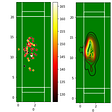## Learning the Clustering Algorithms through Hands-on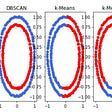## stats.gallery Is Your Friendly Guide to the NEAR Ecosystem## Geospatial big data performance tests with Cosmos DB and data enrichment with Azure Synapse…## Superstore Sales: Explanatory Data Analysis (EDA) and Prediction Models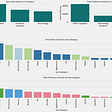## Mean Removal / Standardisation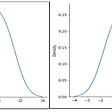## “Night Lights”## Pyth Network

Smarter data for smarter contracts. Pyth is designed to bring real-world data on-chain on a sub-second timescale.

## New Pyth Data Provider: PEAK6## \$AURY The Next Solana Blockchain Game Studio?## Saber announces JSOL-mSOL and JSOL-scnSOL pairs now live!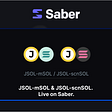## 2022 Proof of Stake Expansion